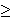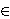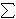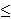# Approximating the Set Cover ProblemI just finished my weekly task of shopping for groceries. This can be a somewhat daunting task because I generally have a list of things that I’ll need which cannot all be purchased at a single location. What often happens is that I find that many of the items on my list are ONLY offered at certain stores – generic brands of certain items for example. My goal then changes from minimizing the total amount of money spent to minimizing the number of stores that I must visit to purchase all of my items.

To formulate this as a mathematical problem, suppose that I have a grocery list of items I would like to buy, represented by the lists item1, item2, …, itemn, where n represents the number of items I have on this list. Suppose also that there are stores Store1, Store2, …, Storem (each one distinct) that offer some combination of items I have on my list. What I would like to do is minimize the number of stores I have to visit to purchase these items.

The problem I just described is famous because it is one that many people face on a regular basis. In a more general form, it is so famous that it has a name for it, called the Set Cover Problem (or the Minimum Set Cover Problem). In the general form of this problem, we replace the grocery list with a set of items called our universe. The lists of items offered at each store are the collections of subsets of the universe. In the problem, as in the example above, we would like to select enough subsets from this collection that we are able to obtain every element in our universe. We would like to do this with as low a number of sets as possible.

In my previous post, I described the 21 problems that Karp proved were NP-Complete. Set Cover was one of those problems, showing that this is a hard problem to solve. What I will do is introduce three ways to reach a near-optimal solution relatively quickly.

Greedy Method

One of the first approaches one may take to solve this problem is to repeatedly select the subset that contains the most new items. That’s how the greedy approach to set cover operates. The method knows to terminate when all elements belong to one of the selected sets. In the shopping example above, this would be accomplished by visiting the store that had the most items on my list and purchasing those items at this store. Once this is done, the items that have been purchased can be crossed off my list and we can visit the store with the most items on my remaining list, stopping when the list is empty.

Linear Programming Relaxation

Instead of stating the set cover problem with words, there is a way of describing the situation with mathematical inequalities. For instance, suppose that the soap I like to purchase is only available at stores Store1, Store4 and Store9. Then I could introduce a variable xi for each store i and the requirement that I purchase this soap can be restated as :

x1 + x4 + x91

Because we can either purchase some items or not purchase these items, each variable xi is 0 or 1 (called a binary variable). We can introduce similar constraints for each element in our universe (or on our grocery list). These inequalities (called constraints) have the form:

for each element eU,i | eSi xi1

Our goal of minimizing the number of sets chosen (stores visited) can be stated by the objective function:
minimize1in xi

So the mathematical formulation for this problem can be stated as

minimize1in xi
Subject to
for each element eU,i | eSi xi1
for each set i, xi{0, 1}.

Formulations of this type, where variables are restricted to a finite set (in this case the x variables being either 0 or 1) are called integer programs. Unfortunately, there is no easy way to solve these formulations either. However, there is a related problem which can be solved quickly.

Instead of restricting the x variables to the values of 0 or 1, we could allow them to take on any value within this range, i.e. 0xi1 for each set Si. Doing this converts the problem from an integer programming problem into a linear programming problem (called the LP-Relaxation), which can be solved quickly. The issue with this method though is that the solution obtained by an LP-Relaxation is not guaranteed to be an integer. In this case, how do we interpret the values xi?

Randomized Rounding Method

One approach to dealing with a non-integer solution to the LP-Relaxation is to treat the xi values as probabilities. We can say that xi is the probability that we select set i. This works because each value of xi is in the range of 0 to 1, which is necessary for a probability. We need to repeatedly select sets with their associated probabilities until all elements in our universe are covered. Selecting our sets based on this procedure is the randomized rounding approach.

Deterministic Rounding Method

A second approach to dealing with a non-integer solution to the LP-Relaxation is to base our solution on the most occurring element. If we let f be this frequency (i.e.the number of sets that the most occurring element occurs in), then we can define a solution by selecting set i if the LP=Relaxation solution gives the variable xi a value of at least (1/f).

None of these three approaches is guaranteed to give an optimal solution to an instance of this problem. I will not go into it in this post, but these can all be shown to be within some guaranteed range of the optimal solution, thus making them approximation algorithms.

Hope you enjoy.

# The Simplex Method

I just added a script which generates a random linear programming problem and executes the Simplex Method Method on it.

The Simplex Method, originally discovered by George Dantzig, is one of the most important algorithms in computer science. It literally connects computer scientists to problems in mathematics, engineering, business, economics, transportation, and a host of problems that are faced by everyday people. For many of these problems, no one has yet discovered a pattern or a simpler means of solving it, other than the Simplex Method. Each of these problems has its own difficulties that distinguish it from other problems. While some such problems are important enough to receive their own studies to improve upon the efficiency of the simplex method (Network Flow problems come to mind), there are just too many problems for each one to receive such consideration. Thus we see the importance of the Simplex Method because it can solve these problems as long as they can be formulated as linear programming problems.

To understand the power of the Simplex Method, we need to understand a class of problems: linear programming problems. A linear programming problem is a problem where we seek to minimize/maximize a linear objective function (over finitely many variables) subject to a finite set of linear inequality constraints over these variables. A general example of a linear programming problem is “The Transportation Problem”.

The Transportation Problem Suppose you own a manufacturing company and its your job to ship your product from warehouse locations all across the United States to a number of customers. Each customer has ordered a certain quantity of the item, and each warehouse can supply up to a certain amount. Then we define the following variables:

m = the number of warehouses
n = the number of customers
ai = the total amount of item available at warehouse i
bj the total requirement of customer j
xi, j = the amount of item shipped from warehouse i to customer j.

In order for things to work properly, we need that the total amount in supply is equal to the the total amount ordered. If we assumed that we have 3 customers and 2 warehouses, then the following constraints help formulate this problem.

x1, 1 + x1, 2 + x1, 3 = a1
x2, 1 + x2, 2 + x2, 3 = a2
x1, 1 + x2, 1 = b1
x1, 2 + x2, 2 = b2
x1, 3 + x2, 3 = b3

You also know the cost of shipping one unit of the item from warehouse i to customer j and label it ci, j.

Then the objective function which we seek to minimze is c1, 1x1, 1 + c1, 2x1, 2 + c1, 3x1, 3 + c2, 1x2, 1 + c2, 2x2, 2 + c2, 3x2, 3.

And we also have that xi, j is nonnegative since we are shipping (and not receiving) goods.

Because we can state this problem as a linear programming problem, we can solve it using the simplex method. Although this problem was very simple to formulate, the ability to formulate a problem as a linear programming problem is seen as a great accomplishment because there exists so much literature on linear programming problems, and a method on how to solve them, (i.e. the Simplex method). For this reason, much work is often dedicated to finding good linear programming representations of problems.

Another set of problems of interest are integer programming problems. These problems add the additional requirement to linear programming problems that some or all of these variables can only take on a discrete set of values instead of a continuous realm. Integer programming problems are generally thought to be more difficult to solve than linear programming problems. What makes integer programming problems special is the fact that many problems that are known to be just as difficult as integer programming problems can be formulated as integer programming problems. Although the Simplex Method does not promise to always solve these problems to optimality, it offers a way to approach integer programming problems, and thus every problems that is known to be just as hard these problems which is at least a starting point.